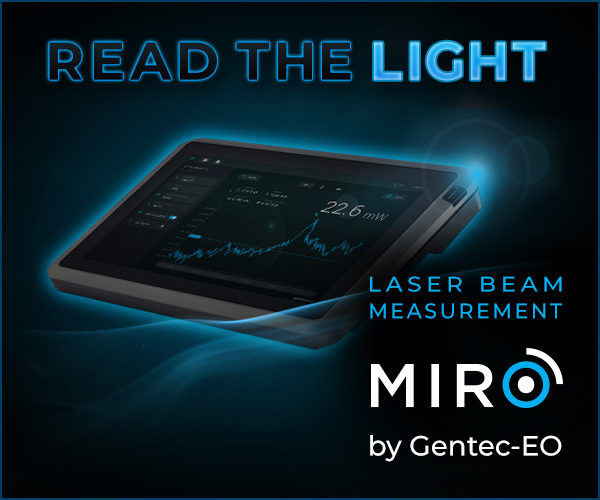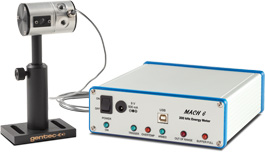EN

# How to calculate laser pulse energy

Facing a hard time calculating laser pulse energy? Or maybe you graduated from university decades ago and simply can’t remember? Don’t worry, we’ll cover the essentials in this blog post.

## The laser pulse energy calculation explained, with numerical examples

If you are trying to calculate the amount of energy that is contained in your laser pulses, you are either working with a pulsed laser and want to know the energy in each individual pulse, or with a CW or pulsed laser that is fired for a known and finite amount of time.

Don’t worry, in both cases, the equations are really simple:

For a pulsed laser, you will need to divide the average power of your source by its repetition rate. Laser specification sheets usually give these two parameters, but for a better accuracy, they can also be measured by suitable instruments.

For example, a Gentec-EO laser power meter can be used to measure average power.

Pulsed laser: Pulse Energy (Joules) = Average Power (Watts) / Repetition Rate (Hertz)

Let’s put some real values in there and assume that you are working with a laser that has a fixed 200 W output and a repetition rate that can be tuned from 20 Hz to 1 kHz. If you set the control at 20 Hz, we have 200 W / 20 Hz = 10 J per pulse.

In order to provide a constant 200 W output with 20 pulses being fired each second, then each of your pulses has to contain 10 J of energy.

If you set the control at 1 kHz (1000 pulses every second), then we have 200 W / 1000 Hz = 0.2 J = 200 mJ in each pulse. For a fixed average power, the higher the repetition rate, the lower the energy per pulse.

For a CW or pulsed laser that is fired for a known and finite amount of time, you will need to multiply the average power of your source by that time, which is often called “Pulse Duration”.

As mentioned previously, the average power of your source is usually something that is given in its specification sheet.Gentec-EO's high-accuracy laser beam measurement instruments help engineers, scientists and technicians in all sorts of laser applications from the factory to the hospital, laboratory and research center. Learn about our solutions for these measurement types:

As for the amount of time that you are going to fire your laser, well, for obvious reasons, the specification sheet does not know that, unless the laser has pre-programmed pulse durations that you can use.

CW or pulsed laser: Pulse Energy (Joules) = Average Power (Watts) * Pulse Duration (Seconds)

Again, let’s use some real values and assume you are working with a CW laser that outputs 500 W. If you fire this laser at an object for exactly 5 seconds, then you have 500 W * 5 s = 2500 J.

This is the total amount of energy that you directed towards this object during this long pulse.

Another example: if your 500 W laser has a 200 ms pre-programmed pulse duration, that will give you 500 W * 0.2 s = 100 J of total energy. The total energy can be controlled by modulating the pulse width/duration.

## Why is pulse energy calculation / measurement important?

Well, if you are reading this article about how to calculate laser pulse energy, you probably need to do it and thus know better than me why this is important for you!

For those who are reading out of curiosity, here is a real-life example: In several medical and surgery applications, pulsed lasers are used treat and cut many types of body tissues.

In order to avoid permanent damage to one’s body (pulse energy higher than what is required) and on the other side avoid incomplete or incorrect treatment (pulse energy lower than what is required), it is mandatory to measure pulse energy to make sure it is stable around the desired sweet spot.

This will ensure both safety and efficiency during treatments.

## What is much better than calculating your laser pulse energy?

The answer is simple, measure it using a Gentec-EO laser joulemeter!If you calculate your pulse energy using average power and repetition rate specifications, you might come close to the real value (what your laser really outputs), but you are more likely to find something that is quite far from it. Why?

The main reason is simple. Your laser does not necessarily output the exact average power that is written in its specification sheet. Same thing for the repetition rate.

In addition, the average power can vary significantly through time with the aging of the laser. What you get on day one can be much higher than what you will get five years after.

You can avoid all this uncertainty by directly measuring your pulses’ energy with a joulemeter. That will give you the best possible accuracy. Gentec-EO offers a very wide range of pyroelectric, photodiodes and thermopiles detectors in order to cover all applications and all laser specifications.